Question

# Consider the following: A student mixes 5.00 mL 2.00 × 10−3 M Fe(NO3)3 with 3.00 mL...

Consider the following:

A student mixes 5.00 mL 2.00 × 10−3 M Fe(NO3)3 with 3.00 mL 2.00 × 10−3 M KSCN. She finds that

in the equilibrium mixture the concentration of FeSCN2+ is 1.28 × 10−4 M. Find Kc for the reaction

Fe3+(aq) + SCN−(aq) ↔ FeSCN2+(aq).

a. What is the initial concentration of Fe3+ in the reaction mixture?

[Fe3+] = ___ x 10-3 M

b. What is the initial concentration of SCN- in the reaction mixture?

[SCN-] = ___ x 10-4 M

c. We can construct an ICE table to help us organize the concentrations of the reactants and products and help us to calculate the equilibrium constant. Based on the first two calculations and the equilibrium concentration of FeSCN2+ ([FeSCN2+] = 0.000128 M), the ICE looks as follows:

 [Fe3+] [SCN-] [FeSCN2+] Initial 0.00125 M 0.00075 M 0 Change -x -x +x Equilibrium 0.00125 M - x 0.00075 M - x 0.000128 M

Based on this, what is the value of "x"?

x = ___ M

d.   What is the equilibrium concentration of Fe3+ And SCN-??

[Fe3+] = ___ M [SCN-] = ___ M

e.   What is the value of the equilibrium constant for the reaction?

Kc = ___ M-1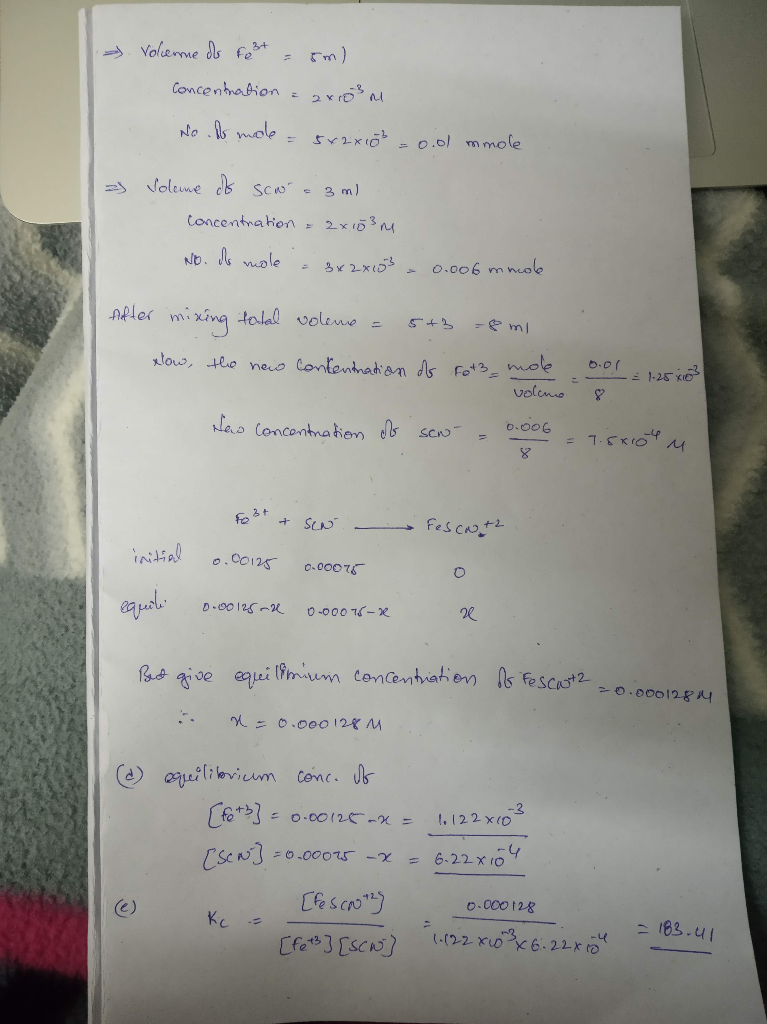#### Earn Coins

Coins can be redeemed for fabulous gifts.

Similar Homework Help Questions
• ### 1. A student mixes 5.00 mL of 2.00 x 10 M Fe(NO3)3 with 5.0 mL of...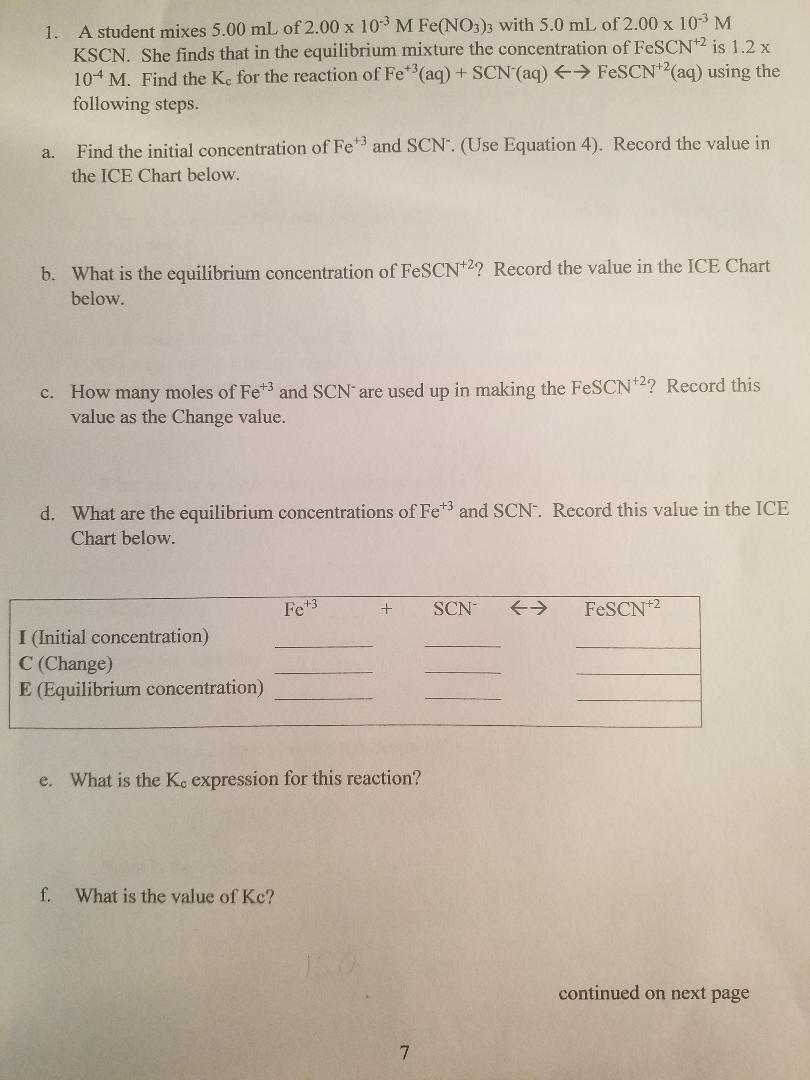1. A student mixes 5.00 mL of 2.00 x 10 M Fe(NO3)3 with 5.0 mL of 2.00 x 10-3 M KSCN. She finds that in the equilibrium mixture the concentration of FeSCN+2 is 1.2 x 104 M. Find the Kc for the reaction of Fe (aq) + SCN (aq) → FeSCN2(aq) using the following steps. a. Find the initial concentration of Fe and SCN. (Use Equation 4). Record the value in the ICE Chart below. b. What is the equilibrium...

• ### A student mixes 5.00 mL 4.00 x 10-3 M Fe(NO3)3 with 5.00 mL 4.00 x 10-3 M KSCN.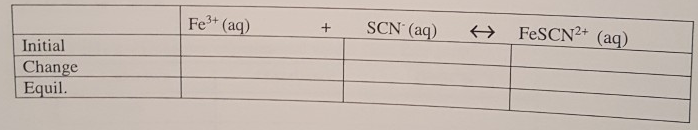A student mixes 5.00 mL 4.00 x 10-3 M Fe(NO3)3 with 5.00 mL 4.00 x 10-3 M KSCN. The student finds that the equilibrium concentration of FeSCN2+ is 5.6 x 10-4M. a. Set up the Kc expression for the following equation. Fe3+ + SCN-  ↔ FeSCN2+b. What is the total volume of the solution? _______ mL c. Fill in the following table for the moles of each species.d. Find the concentration for the above ions. e. Calculate Kc for the reaction.

• ### A student mixes 5.12 mL of 4.02 x 10 M Fe(NO3)3 with 4.88 mL 2.01 x...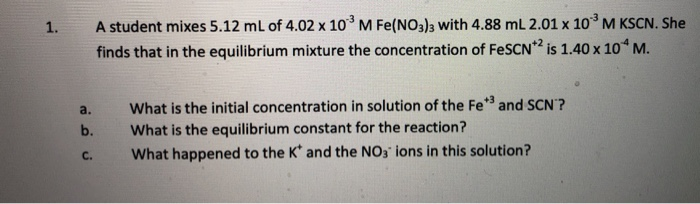A student mixes 5.12 mL of 4.02 x 10 M Fe(NO3)3 with 4.88 mL 2.01 x 103 M KSCN. She finds that in the equilibrium mixture the concentration of FeSCN2 is 1.40 x 10 M. 1. What is the initial concentration in solution of the Fe3 and SCN'? What is the equilibrium constant for the reaction? What happened to the K' and the NO3 ions in this solution? a. b. C. A student mixes 5.12 mL of 4.02 x 10...

• ### A student mixes 5.00 mL of 2.00 times 10^-3 M Fe(NO_3)_3 with 5.00 mL of 2.00...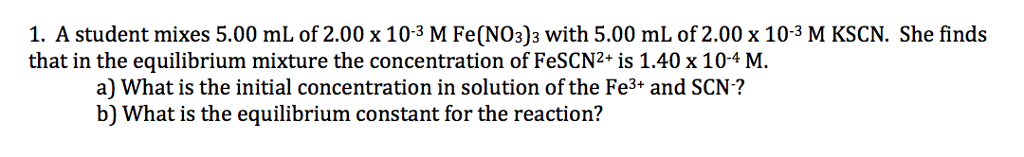A student mixes 5.00 mL of 2.00 times 10^-3 M Fe(NO_3)_3 with 5.00 mL of 2.00 times 10^-3 M KSCN. She finds that in the equilibrium mixture the concentration of FeSCN2^+ is 1.40 times 10^-4 M. a) What is the initial concentration in solution of the Fe^3+ and SCN^-? b) What is the equilibrium constant for the reaction?

• ### 4.81 mL of 0.00200 M Fe(NO3)3, 2.85 mL of 0.00200 M KSCN and 2.34 mL of...

4.81 mL of 0.00200 M Fe(NO3)3, 2.85 mL of 0.00200 M KSCN and 2.34 mL of distilled water were mixed. The resulting solution was allowed to attain equilibrium at 24 oC. The absorbance of the equilibrium solution was recorded, and the [FeSCN2+] was determined graphically to be 7.58 x 10-5 M. Part A: Calculate the number of moles of Fe3+ initially added to the solution. Part B: Calculate the number of moles of FeSCN2+ formed in the solution at equilibrium....

• ### A 25.0 mL volume of 0.0200 M Fe(NO3)3 is mixed with 50.0 mL of 0.00200 M...

A 25.0 mL volume of 0.0200 M Fe(NO3)3 is mixed with 50.0 mL of 0.00200 M NaSCN and 25.0 mL of 0.100 HNO3. The blood-red FeSCN2+ ion forms and the equilibrium is established: Fe3+(aq) + SCN-(aq) <---> FeSCN2+(aq) The equilibrium concentration of FeSCN2+ ([FeSCN2+]) was measured spectrophotometrically and found to be 7.0 x 10-4 mol/L. To calculate the equilibrium constant (Kc) for thr equilibrium system, proceed through the following steps: A. Moles of Fe3+, initial B. Moles of SCN-, initial...

• ### 4. When 5.00 mL of 0.500 M Fe (NO3)3 are mixed with 5.00 mL of 0.250...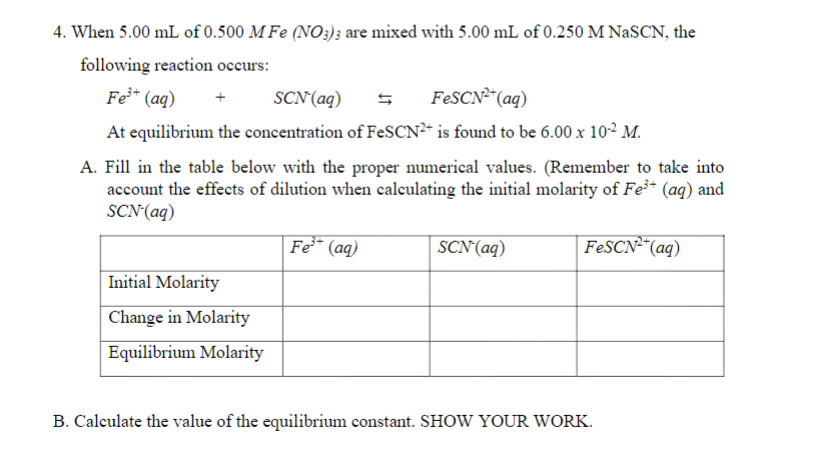4. When 5.00 mL of 0.500 M Fe (NO3)3 are mixed with 5.00 mL of 0.250 M NaSCN, the following reaction occurs: Fe3+ (aq) + SCN(aq) \$ FeSCN2+(aq) At equilibrium the concentration of FeSCN2- is found to be 6.00 x 10-2 M. A. Fill in the table below with the proper numerical values. (Remember to take into account the effects of dilution when calculating the initial molarity of Fe3+ (aq) and SCN'(aq) Fes- (aq) SCN (aq) FeSCN-(aq) Initial Molarity Change...

• ### Use the following procedure for answering questions given below: 3.00 mL of 2.00 x 10' M...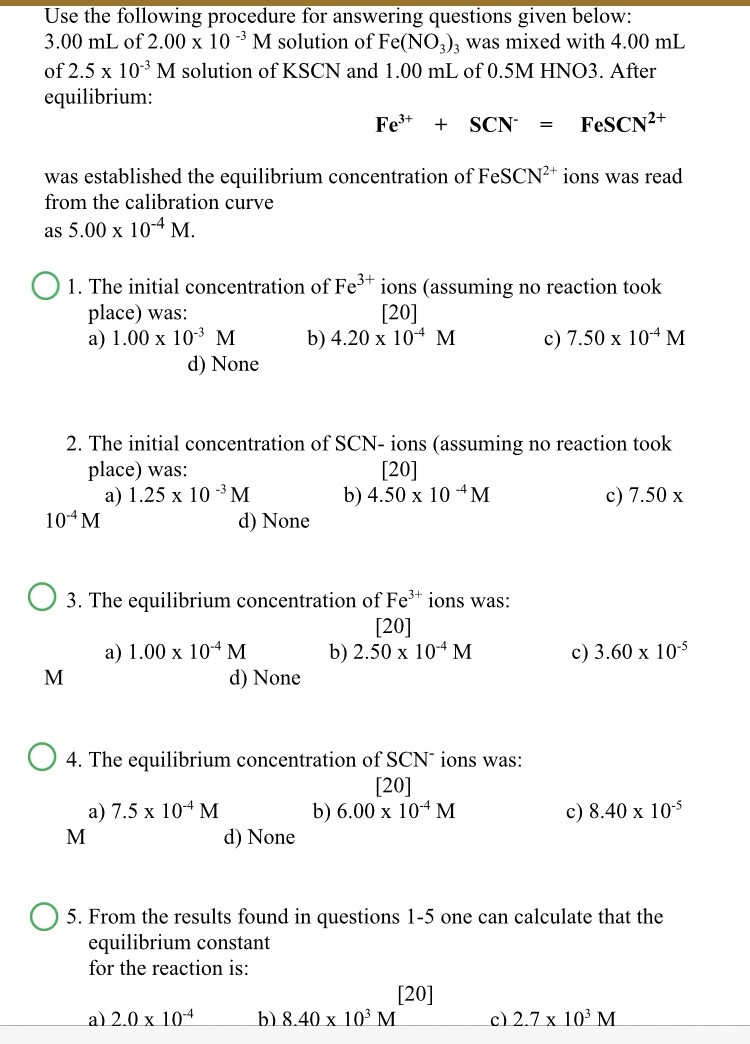Use the following procedure for answering questions given below: 3.00 mL of 2.00 x 10' M solution of Fe(NO3)3 was mixed with 4.00 mL of 2.5 x 10-3 M solution of KSCN and 1.00 mL of 0.5M HNO3. After equilibrium: Fe3+ + SCN FeSCN2+ = was established the equilibrium concentration of FeSCN2+ ions was read from the calibration curve as 5.00 x 10-4 M. 01. The initial concentration of Fe3+ ions (assuming no reaction took place) was:  a) 1.00...

• ### A standard solution of FeSCN2+ is prepared by combining 9.00 mL of 0.200 M Fe(NO3)3 w/1.00 mL of 0.0020 M KSCN

A standard solution of FeSCN2+ is prepared by combining 9.00 mL of 0.200 M Fe(NO3)3 w/1.00 mL of 0.0020 M KSCN. The equilibrium concentration of FeSCN2+ ([FeSCN2+]std) for this standard solution is assumed to be M. What permits this assumption? A. The concentration of Fe(NO3)3is much higher than the concentration of SCN–. B. The equilibrium reaction has a very high Kc. C. The reaction quotient (Q) is greater than Kc. D. The excess Fe3+ prevents the formation of the neutral...

• ### 5.00 mL of 5.00 × 10-1M Fe(NO3)3 are mixed with 2.00 mL of 1.00 x 10-4M NASCN and 3.00 mL of 0.5M HNO3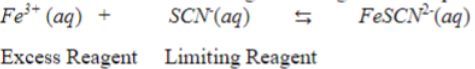5.00 mL of 5.00 × 10-1M Fe(NO3)3 are mixed with 2.00 mL of 1.00 x 10-4M NASCN and 3.00 mL of 0.5M HNO3. A. Calculate the molarity of the Fe3+ in the diluted solution. B. Calculate the molarity of the SCN in the diluted solution. C. Assume that the following reaction goes to completion: Calculate the molarity of the FeSCN2+ in the diluted solution.